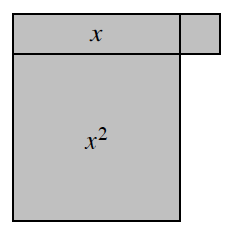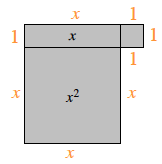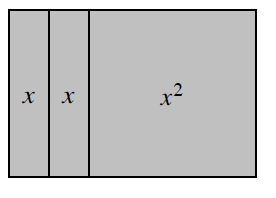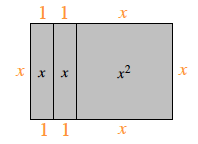### Home > MC2 > Chapter 3 > Lesson 3.1.2 > Problem3-20

3-20.

Copy the diagrams of algebra tiles below on your paper. Then find the perimeter of each shape.

1.The perimeter is the sum of all the sides. What is the value of each side?

Label each side piece.Now, add up all of the sides to find the perimeter.

$x+1+x+1+1+1+x+x$

Simplify to get $4x+4$.

1.Refer to part (a).

Label each side.